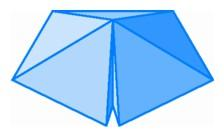The Regular 600-Cell and Its DualFive tetrahedra around an edge in three-space.

But in four-space there is a surprise. We have found three tetrahedra around an edge, and four, and we know that there cannot be six. What about five? It turns out that the dihedral angle of a tetrahedron is just small enough that we can fit five tetrahedra around an edge with a very small amount of room to spare, which allows for folding into four-space.

This configuration of five tetrahedra around each edge is found in a four-dimensional polytope having 600 tetrahedra and therefore going by the name of a 600-cell. We can describe the part of this polytope near a vertex by using the same construction we used to get from a pentagon to the pentagonal pyramid at each vertex of a regular icosahedron. For the icosahedron, we start with a regular pentagon in the plane and choose a point above its center so that the distance to each of the five vertices equals the length of the original edges. For the 600-cell, we begin with an icosahedron in three-space and choose a point "above" its center in four-space so that the distance to each of the 12 vertices equals the length of the original edges. Drawing the edges from this point to the original vertices forms 20 tetrahedra coming together at the vertex of the 600-cell. Once again, we cannot actually carry out this construction without access to a fourth direction perpendicular to our space, but the procedure is clear.

Every regular polytope should have a dual, and this dual will be another regular polytope. Since each vertex of the 600-cell is surrounded by 20 tetrahedra, each cell of the dual polytope will have 20 vertices. Thus the dual cells will be dodecahedra. It may seem surprising that a regular polytope can be built out of dodecahedra since three regular dodecahedra would have to fit around an edge in three-dimensional space. Yet indeed they do fit. The dihedral angle of a dodecahedron turns out to be slightly less than one-third of a complete rotation, so three of them fit around an edge with a small amount of room left over. The dual of the 600-cell turns out to have 120 regular dodecahedra, leading to its name, the 120-cell.

We had five regular polyhedra in three-space, and the above analysis has already produced five regular polytopes in four-space. We don't get any new examples by using icosahedra since the dihedral angle at an edge of a regular icosahedron is larger than one-third of a full rotation. But still remaining is another possible building block, the octahedron, and it provides one more surprise.